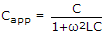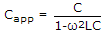# Materials and Components Section 1

36. Assertion (A): At a temperature of 1000 K, thermionic emission current is about 0.1 A/cm2 of surface.

Reason (R): Thermionic emission current is given by Ith = SA0 T2 e-Ew/kT.

 A. Both A and R are true and R is correct explanation of A B. Both A and R are true but R is not correct explanation of A C. A is true but R is false D. A is false but R is true

Explanation:

If different values are substituted in the equation for Ith the value of emission current comes out to be about 0.1 A/cm2.

37. At room temperature, the current in intrinsic semiconductor is due to
 A. holes B. electrons C. ions D. holes and electrons

Explanation:

Both electrons and holes contribute to flow of current in semiconductors.

38. Ferroelectric materials are those which
 A. cannot be polarized B. have a permanent polarization C. have ae equal to zero D. have μp = 0

Explanation:

Ferroelectric materials have permanent polarization.

39. Assertion (A): Power loss in a conductor of resistance P = I2R.

Reason (R): When a conductor is carrying current with current density J as a result of applied field E, then heat developed/m3/ second = JE.

 A. Both A and R are true and R is correct explanation of A B. Both A and R are true but R is not correct explanation of A C. A is true but R is false D. A is false but R is true

Explanation:

Heat developed depends an power loss.

40. Assertion (A): For Diamond, μr = 1

Reason (R): Diamond is a diamagnetic material.

 A. Both A and R are true and R is correct explanation of A B. Both A and R are true but R is not correct explanation of A C. A is true but R is false D. A is false but R is true

Explanation:

For diamagnetic materials μr = 1.

41. If n denotes principal quantum number, the angular quantum number l = 0, 1… (n – 1).
 A. True B. False

Explanation:

Angular quantum number determines the angular momentum of electron.

42. Assertion (A): In a perfect capacitor, the current density is given by ω ∈0E0rcos(ωt + 90°), where ∈r is real part of dielectric constant.

Reason (R): In a perfect capacitor, dielectric losses are zero.

 A. Both A and R are true and R is correct explanation of A B. Both A and R are true but R is not correct explanation of A C. A is true but R is false D. A is false but R is true

Explanation:

If dielectric losses are zero, the current in capacitor leads the applied voltage by 90°.

Also imaginary part of is ∈r is zero.

43. Assertion (A): For a solenoid having N turns,.

Reason (R): For all magnetic materials, B increases linearly with I.

 A. Both A and R are true and R is correct explanation of A B. Both A and R are true but R is not correct explanation of A C. A is true but R is false D. A is false but R is true

Explanation:

Increase of B with I is not linear. Hence R is false.

44. Assertion (A): From the slope of the line in Figure, we can find permanent dipole moment of the molecules.

Reason (R): Total polarization of a polyatomic gas is given by P = N(ae + ai + μ2p / 3kT)E.A. Both A and R are true and R is correct explanation of A B. Both A and R are true but R is not correct explanation of A C. A is true but R is false D. A is false but R is true

Explanation:

The slope of the line in figure is.

Since the total polarization is as given by the expression, we can find the permanent dipole moment of the molecules from the slope.

45. Assertion (A): If E is available energy state eV and EF is Fermi level, then probability of energy state E being occupied = 0.5.

Reason (R):.

 A. Both A and R are true and R is correct explanation of A B. Both A and R are true but R is not correct explanation of A C. A is true but R is false D. A is false but R is true

Explanation:

E = EF, f(E) = 0.5.

46. If a piece of wood is placed in a magnetic field
 A. the magnetic field will not be affected B. the magnetic lines of force will bend towards the piece C. the magnetic lines of force will bend away from the piece D. the flux may increase or decrease

Explanation:

Wood is non-magnetic.

47. The apparent capacitance at a high angular frequency ω of an air cored capacitor having capacitance C and inductance L is given by
 A.B.C.D.Explanation:

The combination can be represented as L and C in series..

Therefore,48. The number of valence electrons in donor impurity are
 A. 5 B. 4 C. 3 D. 1

Explanation:

Donor impurity has 5 valence electrons (while silicon has 4 valence electrons).

Hence donor impurity can donate electrons.

49. In a true valence crystal, the bonding between the atoms is accomplished by the sharing of valence electrons.
 A. True B. False

Explanation:

Sharing of valence electons is a characteristic of true valence crystal.

50. Which of the following are donor impurities?

1. Gold
2. Phosphorus
3. Boron
4. Antimony
5. Arsenic
6. Induim

Select the correct answer using the following codes

 A. 1, 2, 3 B. 1, 2, 4, 6 C. 3, 4, 5, 6 D. 2, 4, 5